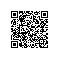# 日志服务IPython/Jupyter扩展实战：下载数据为Excel文件## 问题

{"city": "123", "province": "vvv"}
{"city": "shanghai", "pop": "2000"}
{"name": "xiao ming", "home": "shanghai"}

## 前提

### 安装Excel相关组件

pip install openpyxl xlrd xlwt XlsxWriter
• openpyxl - 用于Excel 2010 xlsx/xlsm文件的读写
• xlrd - 读取Exce (xls格式）
• xlwt - 写Excel (xls格式）
• XlsxWriter - 写Excel (xlsx)文件

## 场景

### 1. 将结果保存到Excel中

%%log -1day ~ now
* | select date_format(date_trunc('hour', __time__), '%H:%i') as dt,
count(1)%100 as pv,
round(sum(if(status < 400, 1, 0))*100.0/count(1), 1) AS ratio
group by date_trunc('hour', __time__)
order by dt limit 1000
df1 = log_df
df1.to_excel('output.xlsx')

### 2. 将结果保存到Excel多个Sheet中

import pandas as pd
writer = pd.ExcelWriter('output2.xlsx')

df1.to_excel(writer, sheet_name='data1')
df2.to_excel(writer, sheet_name='data2')

writer.save()

### 3. 定制Excel细节格式

Pandas默认使用Xlwt模块xls文件、使用Openpyxl模块xlsx文件。而使用XlsxWriterxlsx功能更加全面灵活，但需要如下配置。

writer = pd.ExcelWriter('output2.xlsx', engine='xlsxwriter') 

### 4. 其他格式

Pandas DataFrame还可以保存其他格式，例如csvhtml等，可以进一步参考这里

# 进一步参考